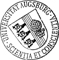## Modulation Equation for SPDEs in Unbounded Domains With Space-Time White Noise - Linear Theory

• We study the approximation of SPDEs on the whole real line near a change of stability via modulation or amplitude equations. Due to the unboundedness of the underlying domain a whole band of infinitely many eigenfunctions changes stability. Thus we expect not only a slow motion in time, but also a slow spatial modulation of the dominant modes. As a first step towards a full theory of modulation equations for nonlinear SPDEs on unbounded domains, we focus, in the results presented here, on the linear theory for one particular example, the Swift-Hohenberg equation only. These linear results are one of the key technical tools to carry over the deterministic approximation results to the stochastic case with additive forcing. One technical problem for establishing error estimates arise from the spatially translation invariant nature of space-time white noise on unbounded domains, which implies that at any time due to noise we can expect that the error is somewhere in space always veryWe study the approximation of SPDEs on the whole real line near a change of stability via modulation or amplitude equations. Due to the unboundedness of the underlying domain a whole band of infinitely many eigenfunctions changes stability. Thus we expect not only a slow motion in time, but also a slow spatial modulation of the dominant modes. As a first step towards a full theory of modulation equations for nonlinear SPDEs on unbounded domains, we focus, in the results presented here, on the linear theory for one particular example, the Swift-Hohenberg equation only. These linear results are one of the key technical tools to carry over the deterministic approximation results to the stochastic case with additive forcing. One technical problem for establishing error estimates arise from the spatially translation invariant nature of space-time white noise on unbounded domains, which implies that at any time due to noise we can expect that the error is somewhere in space always very large.Author: Luigi Amedeo Bianchi, Dirk BlömkerORCiDGND urn:nbn:de:bvb:384-opus4-32139 https://opus.bibliothek.uni-augsburg.de/opus4/3213 Preprints des Instituts für Mathematik der Universität Augsburg (2015-08) Preprint English 2015 Universität Augsburg 2015/07/28 modulation equation; amplitude equation; stochastic convolution; Gaussian processes; random fields Modulationsgleichung; Amplitudengleichung; Faltung ; Gauß-Prozess; Zufälliges Feld; Stochastische partielle Differentialgleichung `Erschienen in Stochastic Processes and their Applications, 126, 10, S. 3171-3201, https://doi.org/10.1016/j.spa.2016.04.024` Mathematisch-Naturwissenschaftlich-Technische Fakultät Mathematisch-Naturwissenschaftlich-Technische Fakultät / Institut für Mathematik 5 Naturwissenschaften und Mathematik / 51 Mathematik / 510 MathematikDeutsches Urheberrecht mit Print on Demand